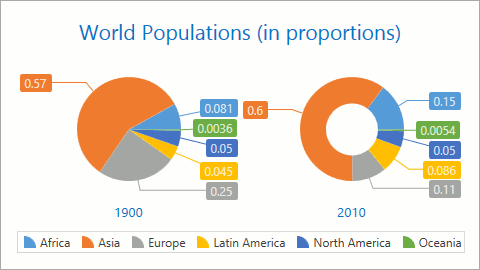20.1
19.2
19.1
18.2
18.1
17.2
The page you are viewing does not exist in version 17.2. This link will take you to the root page.

# Simple 2D Diagram

The document highlights the Simple 2D Diagram's specifics. This diagram displays series such as Pies and Donuts in a two-dimensional view.This document consists of the following sections:

## Compatible Series Types

The diagram can only contain series of compatible types. You can add the following series to the Simple 2D Diagram:

Series type Image
Pie and DonutNested DonutFunnel## How to Create a Chart with a Simple Diagram

The markup below demonstrates how to design a chart with a simple diagram that contains two pie series.

``````<dxc:ChartControl>
<!-- Simple diagram settings. -->
<dxc:SimpleDiagram2D Dimension="2"
LayoutDirection="Vertical">
<!-- The first Pie series settings. -->
<dxc:PieSeries2D>
<dxc:SeriesPoint Argument="A" Value="6"/>
<dxc:SeriesPoint Argument="B" Value="11"/>
<dxc:SeriesPoint Argument="C" Value="15"/>
</dxc:PieSeries2D>
<!-- The second Pie series settings. -->
<dxc:PieSeries2D>
<dxc:SeriesPoint Argument="A" Value="10"/>
<dxc:SeriesPoint Argument="B" Value="7"/>
<dxc:SeriesPoint Argument="C" Value="12"/>
</dxc:PieSeries2D>
</dxc:SimpleDiagram2D>
</dxc:ChartControl>
``````

The markup uses the following classes and properties:

Class or Property Description
ChartControl The Chart control.
SimpleDiagram2D The 2D Simple diagram.
SimpleDiagram2D.Dimension Specifies the number of pie charts that can be in one line (row or column).
SimpleDiagram2D.LayoutDirection Specifies how separate series are relative to each other (horizontally or vertically).
PieSeries2D A pie series.
SeriesPoint A series point.
SeriesPoint.Argument A series point's argument.
SeriesPoint.Value A series point's value.

You can also access a 2D Simple Diagram at runtime using the following code:

``````// Cast Diagram to the SimpleDiagram2D type.
SimpleDiagram2D diagram = chart.Diagram as SimpleDiagram2D;
if (diagram != null) {
// Access the diagram's options.
diagram.Dimension = 2;
diagram.LayoutDirection = LayoutDirection.Vertical;
}
``````